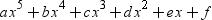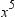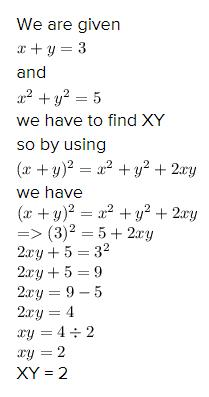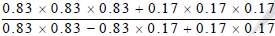Courses

# Test: Polynomials - 1

## 25 Questions MCQ Test Mathematics (Maths) Class 9 | Test: Polynomials - 1

Description
This mock test of Test: Polynomials - 1 for Class 9 helps you for every Class 9 entrance exam. This contains 25 Multiple Choice Questions for Class 9 Test: Polynomials - 1 (mcq) to study with solutions a complete question bank. The solved questions answers in this Test: Polynomials - 1 quiz give you a good mix of easy questions and tough questions. Class 9 students definitely take this Test: Polynomials - 1 exercise for a better result in the exam. You can find other Test: Polynomials - 1 extra questions, long questions & short questions for Class 9 on EduRev as well by searching above.
QUESTION: 1

### A cubic polynomial is a polynomial with degree

Solution:

A cubic polynomial is a polynomial of degree 3. A univariate cubic polynomial has the form f(x) = a3 x3 + a2 x2 + a1 x + a0. An equation involving a cubic polynomial is called a cubic equation. A closed-form solution known as the cubic formula exists for the solutions of an arbitrary cubic equation.

QUESTION: 2

### A polynomial of degree 5 in x has at most

Solution:

A polynomial of degree 5 is of the form p(x) =, where a, b, c, d, e, and f are real numbers and a ≠ 0.

Thus, p(x) can have at most 6 terms and at least one term containingQUESTION: 3

### The coefficient of x3 in the polynomial 5 + 2x + 3x2 – 7x3 is

Solution:
QUESTION: 4

The quadratic polynomial whose sum of zeroes is 3 and the product of zeroes is –2 is :

Solution:

Sum of zeros = 3/1
-b/a = 3/1 .....................(1)

Product of zeros = -2/1
c/a = -2/1 ...................(2)

From equation (1) and (2)
a = 1
-b = 3, b = -3
c = -2

ax^2+by+c
= x2-3x-2

QUESTION: 5

A linear polynomial :-

Solution:

A number of zeroes of an n -degree polynomial = n.

First, a linear polynomial is in the form of ax + b, a≠0, a,b ∈R

The degree of the polynomial = highest degree of the terms
So here the highest degree is 1.

Hence, Linear polynomial has only one zero.

QUESTION: 6

If x + y = 3, x2 + y2 = 5 then xy is

Solution:QUESTION: 7

When the polynomial x3 + 3x2 + 3x + 1 is divided by x + 1, the remainder is :-

Solution:

The zero of x + 1 is –1

And by remainder theorem, when

p(x) = x3 + 3x2 + 3x + 1 is divided by x + 1, then remainder is p(–1).

∴ p(–1) = (–1)3 + 3 (–1)2 + 3(–1) + 1

= –1 + (3 × 1) + (–3) + 1

= –1 + 3 – 3 + 1

= 0

Thus, the required = 0

QUESTION: 8

If the polynomial 2x3 – 3x2 + 2x – 4 is divided by x – 2, then the remainder is :-

Solution:
QUESTION: 9

The value of k for which x – 1 is a factor of the polynomial 4x3+ 3x2 – 4x + k is :-

Solution:

X - 1 is a factor of 4x3 + 3x2 -4x +k
then x=1 is one root of 4x3 + 3x2 -4x +k

put x= 1
4x3 +3x2 -4x +k = 0

=> 4 (1)3 +3 (1)2-4 (1) +k =0

=> 4 + 3 - 4 + k = 0

=> k = -3

QUESTION: 10

The value of k for which x + 1 is a factor of the polynomial x3 + x2 + x + k is :-

Solution:
QUESTION: 11

The value of m for which x – 2 is a factor of the polynomial x4 – x3 + 2x2 – mx + 4 is :-

Solution:
QUESTION: 12

The factors of 2x2 – 3x – 2 are :-

Solution:
QUESTION: 13

If x + 2 is a factor of x3 – 2ax2 + 16, then value of a is

Solution:
QUESTION: 14

The factors of x3 – 2x2 – 13x – 10 are :-

Solution:
QUESTION: 15

The expanded form of (2x – 3y – z)2 is :-

Solution:
QUESTION: 16

The expanded form of (x + y + 2z)2 is :-

Solution:
QUESTION: 17

The expanded form of (x+1/3)3 is :-

Solution:
QUESTION: 18

x3 + y3 + z3 – 3xyz is :-

Solution:

We know that x3 + y3 + z3 – 3xyz = (x + y + z) (x2 + y2 + z2 – xy – yz – zx).
If x + y + z = 0, then x3 + y3 + z3 – 3xyz = 0 or x3 + y3 + z3 = 3xyz.

QUESTION: 19

(a – b)3 + (b – c)3 + (c – a)3 is equal to :-

Solution:

Let x = (a – b), y = (b – c) and z = (c – a)
Consider, x + y + z = (a – b) + (b – c) + (c – a) = 0
⇒ x3 + y3 + z3 = 3xyz
That is (a – b)3 + (b – c)3 + (c – a)3 = 3(a – b)(b – c)(c – a)

QUESTION: 20is equal to :-

Solution:
QUESTION: 21

√2 is a polynomial of degree

Solution:

The highest power of the variable is known as the degree of the polynomial.

√2x^0 = √2
hence the degree of the polynomial is zero.

QUESTION: 22

The degree of the polynomial 4x4+0x3+0x5+5x+74x4+0x3+0x5+5x+7 is

Solution:

The degree of the polynomial 4x4+0x3+0x5+5x+74x4+0x3+0x5+5x+7 is

QUESTION: 23

The degree of the zero polynomial is

Solution:

The degree of zero polynomial is not defined, because, in zero polynomial, the coefficient of any variable is zero i.e., Oxor Ox5, etc. Hence, we cannot exactly determine the degree of the variable.

QUESTION: 24

The value of the polynomial 5x−4x2+3, when x = −1 is

Solution:

Let p (x) = 5x – 4x2 + 3 …(i)
On putting x = -1 in Eq. (i), we get
p(-1) = 5(-1) -4(-1)2 + 3= - 5 - 4 + 3 = -6

QUESTION: 25

If p(x) = x + 3, then p(x) + p(-x) is equal to

Solution:

p(x)=x+3

p(-x)=-x+3

p(x)+p(-x)=x+3-x+3=6